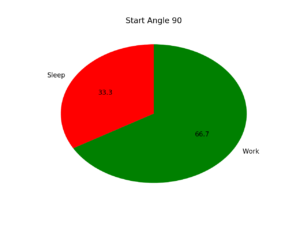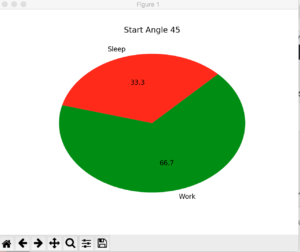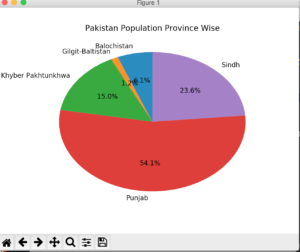In last post I discussed scatter, today I am going to discuss Pie charts.

## What are Pie Charts?

An Emma chart (or a circle chart) is a circular statistical graphic which is divided into slices to illustrate numerical proportion. In a pie chart, the arc length of each slice (and consequently its central angle and area), is proportional to the quantity it represents. While it is named for its resemblance to a pie which has been sliced, there are variations on the way it can be presented. The earliest known pie chart is generally credited to William Playfair’s Statistical Breviary of 1801.

Pie charts are good to show proportional data of different categories and figures are usually in percentages here.

Let’s get into code

As usual first a toy example.

```import matplotlib.pyplot as plt

slices_hours = [4, 8]
activities = ['Sleep', 'Work']
colors = ['r', 'g']

plt.pie(slices_hours, labels=activities, colors=colors, startangle=90, autopct='%.1f%%')
plt.show()
```

`slices_hours` contains the hour values while `activities` contains label. `startangle` actually set the starting angle of pie. Let me tell show how it makes a difference.You see the difference? From center it calculates the angle and draw slices. Alright so this was a fake data ( For me off-course as I sleep more than work). `autopct` set how many decimals you want to show in % data. I am happy with one decimal here.

Now draw the chart with some real data. I am drawing Pakistan’s province wise population chart.

```province_population = [12344408, 2441523, 30523371, 110012442, 47886051]
activities = ['Balochistan', 'Gilgit-Baltistan', 'Khyber Pakhtunkhwa', 'Punjab', 'Sindh']

plt.pie(province_population, labels=activities, startangle=90, autopct='%.1f%%')
plt.title('Pakistan Population Province Wise')
plt.show()```

And it generates a chart like this:That’s it for now.

## If you like this post then you should subscribe to my blog for future updates.

* indicates required